×#### Thank you for registering.

One of our academic counsellors will contact you within 1 working day.

Click to Chat

1800-1023-196

+91-120-4616500

CART 0

• 0

MY CART (5)

Use Coupon: CART20 and get 20% off on all online Study Material

ITEM
DETAILS
MRP
DISCOUNT
FINAL PRICE
Total Price: Rs.

There are no items in this cart.
Continue Shopping```Operations on Sets

Table of Content

Meaning of Operations on Sets

What is the meaning of Sets in Mathematics?

Operations on Sets in Discrete Mathematics

Types of Operations on Sets

What is the Union in Math?

What is the meaning of Intersection of sets?

Difference of Sets

What is Union and Intersection?

Properties of Union and Intersection of Sets

Meaning of Operations on Sets

There are so many operations in math like addition, subtraction, multiplication, division etc. When we use these operations on numbers, we get a new number. Example, if we add 23 with 42, we get a new number as answer i.e. 65. Similarly, when we do some operations on sets we get a new set. To understand operations on sets first we need to understand sets.

What is the meaning of Sets in Mathematics?

A set is a collection of different objects having some common property and all the objects are the elements of set. In mathematics, we use collections of different things like group of natural numbers, even numbers, etc.Let’s see some examples of sets in mathematics;

Set of different shapes

Set of different numbers

Set of animals

Set of flowers

The objects of the group are the elements of a set. We have to list each element separated by a comma, and then put curly brackets around it. These curly brackets { } are also called "braces”. The repetition of elements and the order of elements have no relevance in the set.

Example

A = {1, 3, 5, 7}, A is a set of odd numbers less than 8.

B = {2, 4, 6, 8}, B is a set of even numbers less than 9.

If we do some operation on these sets then it will produce a new set.

Operations on Sets in Discrete Mathematics

Discrete Mathematics is the study of discrete structures to represent discrete objects rather than continuous. Most of the discrete structures are made up of sets.

Example

Combinations

Relations

Graphs

Some important sets in discrete mathematics are:

Natural Numbers

N = {0, 1, 2, 3, …}

Integers

Z = {…, -2, -1, 0, 1, 2, …}

Positive Integers

Z+ = {1, 2, 3.…}

Rational Numbers

Q = {p/q | p ∈ Z, q ∈ Z q ≠ 0}

Real Numbers

Set of all integers including decimal numbers.

Types of Operations on Sets

Basically, there are three operations on sets:

Union of Sets

Intersection of Sets

Difference of Sets

What is the Union in Math?

The union of two sets A and B is the set of all the elements present in A or B or both. We will take the common elements at once only. It is denoted with the symbol “∪” read as “union”.

Symbolically,

A∪B = {x: x ∈A or x ∈B}

Example

X = {a, e, i}

Y = {i, o, u}

X∪Y = {a, e, i, o, u} i.e. X union Y is a new set having all the elements either in X or Y without repeating any element.

The union of two sets can be represented with Venn diagram:

Union of two Joint Sets: The two sets having some common element within them are called the Joint Sets. It is shown by two overlapping circles in Venn diagram.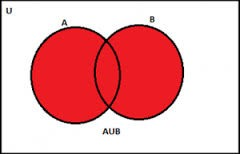Symbolically,

Here,

A∩B ≠ ∅ that is A intersection B is not an empty set.

The red colored portion shows A∪B reads as “A union B”.

It contains all the elements of A and B both.

But here n (A) + n (B) ≠ n(A∪B) because some elements are common and they will be counted twice if we will add like this. So we can say that n (A) +n (B) – n(A∩B) = n(A∪B), where A∩B is the common element within the two sets.

Union of two Disjoint Sets: The two sets which have no common element within them are called Disjoint Sets. This shows by two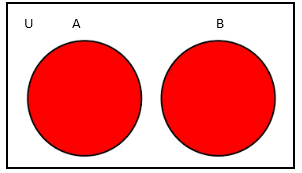separate circles and does not overlap each other.

A∩B = ∅ that is. A intersection B is an empty set.

Here, A and B are two disjoint sets.

In the case of disjoint sets n (A) +n (B) = n(A∪B) as there is no common element within them.

Union of set and its Subset: A set X is a subset of Y if all the elements of X are the elements of Y also. It is represented by “⊆” means “is a subset of”.

Symbolically,

X⊆Y if x ∈ X ⇒ x∈Y

The union of two set X and Y is the set of all the elements belong to both the sets X and Y .If X is the subset of Y then Y contains all the elements of X. So the union of the set and its subset is the set itself.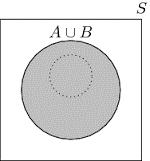Symbolically,

X∪Y = Y⇒ X⊆  Y

X union Y is Y itself implies that X is the subset of Y.

The right picture shows that A∪B = A as B is the subset of A.

Properties of Operations of Union

A∪B = B∪A  (Commutative law)

This says that A∪B and its opposite will always be equal.

A∪(B∪C) = (A∪B)∪C  (Associative law)

The order of operation does not have any relevance here; the result will always be equal.

A ∪ ϕ = A  (Law of identity element, is the identity of ∪)

The union of a set and the empty set will always be the set as the empty set contains zero elements.

A∪A = A  (Idempotent law)

The union of the set with itself will always be the set itself as every set is a subset of itself. And we do not consider the repetition of elements.

U∪A = U (Law of ∪, domination) ∪ is the universal set.

The union of the set and its universal set will always be the universal set as the universal set contains all the elements of A also.

A∪A′ = U  (Negation)

The union of the set and its complement will always be the universal set as both are the subset of universal set and together they contain all the elements of universal set.

What is the meaning of Intersection of Sets?

The intersection of two sets A and B means a set of all the elements which are common to both A and B. It is represented by symbol “∩” reads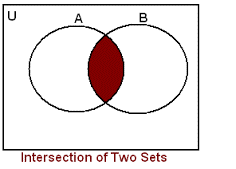as “Intersection”.

A∩B = {x: x x ∈ A and x ∈ B}

The intersection of two sets can be shown with the Venn diagram:

Here the shaded portion shows the common elements between A and B.

“A∩B” reads as “A intersection B”.

Intersection of two disjoint sets will always be empty set as there is no common element within the two disjoint sets.

A∩B = ∅ if A and B are two disjoint sets.

Properties of operations of Intersection

A ∩ B = B ∩ A (Commutative law).

This shows that A∩B is always equal to its opposite.

( A ∩ B ) ∩ C = A ∩ ( B ∩ C ) (Associative law).

The order of operation does not have any relevance here; the result of intersection will always be equal.

φ ∩ A = φ ( law of domination)

The intersection of any set and empty set will always be the empty set as empty set is the subset of all sets.

U ∩ A = A (Law of identity).

The intersection of a set and the universal set will always be the set itself. As every set is the subset of its universal set.

A ∩ A = A (Idempotent law)

The intersection of the set with itself is the set itself.

A∩A′ = ∅ (negation)

The intersection of the set and its complement will always be the empty set as these are two disjoint sets and they have no common element within them.

A ⊆ B if and only if A ∩ B = A.

If A is the subset of B then A intersection B will be A as B contains all the elements of A. So the common elements within them will be all the elements of A.

Difference of Sets

The difference of two sets A and B represents as A-B i.e. all the element which are present in A but not in B.

Symbolically,

A – B = { x : x ∈ A and x ∉ B } and vice versa.

The difference of the sets can be represented by Venn diagram:Here in the above picture two joint sets, A and B are given. There are some common elements within them which are represented by A∩B.

In first picture the green portion shows “A-B” reads as “A minus B” .It means all the elements which belongs to A but not to B. Or you can say that subtract the common elements of A and B from A.

In second picture, green portion shows “B-A” reads as “B minus A” i.e. all the elements which belongs to B but not to A.

A-B is not necessarily equal to B-A.

Example

A = {D, I, A, R, Y}

B = {D, I, G}

A-B = {A, R, Y}

B-A = {G}

A-B ≠ B-A

What is the difference of two Disjoint Sets?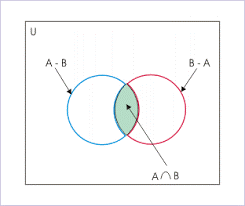If X and Y are two disjoint sets the X-Y will be X only. As there is no common elements within them to subtract from X.

X-Y = X

Y-X = Y

The sets A – B, A ∩ B and B – A are disjoint sets, it means, the intersection of any of these two sets is the empty set that is ∅

What is Union and Intersection?

When there are more than two sets, there relations could be understood by union and intersection.

The major difference between the two are:

Union

Intersection

Definition

The union of two sets A and B is the set of all the elements present in A or B or both.

The intersection of two sets A and B means a set of all the elements which are common to both A and B.

Symbol

∪

∩

Mathematical definition

A∪B = {x: x ∈A or x ∈B }

A∩B = {x:x x ∈ A and x ∈ B}

Example

A = {m,n,o,p}

B = {o,p,q,r}

A∪B = {m,n,o,p,q,r}

A = {m, n, o, p}

B = {o, p, q, r}

A∩B = {o, p}

Venn diagram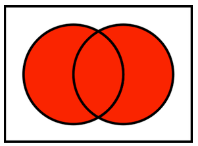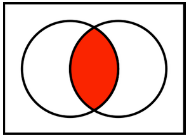Properties of Union and Intersection of Sets

As both the union and intersection of sets have some properties of their own like commutative and associative law but together they follow some more properties like:

The union and intersection together form a Boolean algebra; this is called Distributive Law in which intersection distributes over union and vice versa.

Intersection distributes over union

∪ (B∩C) = (A∪B) ∩ (A∪C)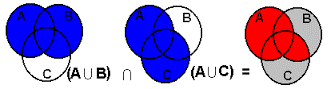Union distributes over intersection

A∩ (B∪C) = (A∩B) ∪ (A∩B)( A ∩B ) ⊆ A and ( A ∩B ) ⊆ B, as all the elements of the intersection of A and B that is. the common elements, are the elements of both A and B,

A ⊆ ( A ∪ B ) and B ⊆ ( A ∪B ) , as A union B is the set contains all the elements of A or B or both.

Operations on Sets
```### Course Features

• 731 Video Lectures
• Revision Notes
• Previous Year Papers
• Mind Map
• Study Planner
• NCERT Solutions
• Discussion Forum
• Test paper with Video Solution